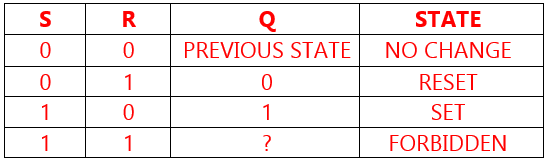# Difference between SR Flipflop and RS Flipflop ?

Flip-flops and latches are used as data storage elements. A flip-flop is a device which stores a single bit (binary digit) of data; one of its two states represents a “one” and the other represents a “zero”.

Also Read : SR Flipflop using PLCFig : Animation of a SR latch

## Difference between SR Flipflop and RS Flipflop ?

The theoretically SR and RS flip-flops are same. When both S & R inputs are high the output is indeterminate.In PLC and other programming environments, it is required to assign determinate outputs to all conditions of the flip-flop. Hence, RS and SR flip-flops were designed.

Let’s see the difference between Set priority flip-flop and Reset priority flip-flop ?

In RS flipflop, Reset input has high priority

In SR flipflop, Set input has high priority.

i.e. When both S & R inputs of the flip flop are high

SR flip flop sets the output. SR (SetRest) flipflop will be SET(1)

while RS flip flop resets the output. RS (Resetset) flipflop will be RESET (0)

Further,

## SR flipflop

• When the result Q = S or (note : not R and Q)
• inputs are marked S1 (priority set input) and R (reset input)
• S = 0 and R = 0 → Q = Q (unchanged)
• S = 0 and R = 1 → Q = 0 (reset)
• S = 1 and R = 0 → Q = 1 (set)
• S = 1 and R = 1 → Q = 1 (set is dominant)

## RS flipflop

• When the result Q = not R and (S or Q)
• inputs are marked R1 (priority reset input) and S (set input)
• S = 0 and R = 0 → Q = Q (unchanded)
• S = 0 and R = 1 → Q = 0 (reset)
• S = 1 and R = 0 → Q = 1 (set)
• S = 1 and R = 1 → Q = 0 (reset is dominant)
Don't Miss Our Updates
Be the first to get exclusive content straight to your email.
We promise not to spam you. You can unsubscribe at any time.

### 3 thoughts on “Difference between SR Flipflop and RS Flipflop ?”

1.SR and RS are fals

•S-R flip flop
S=1,R=1,Q=0
&
R-S flip flop
R=1,S=1,Q=1

2.